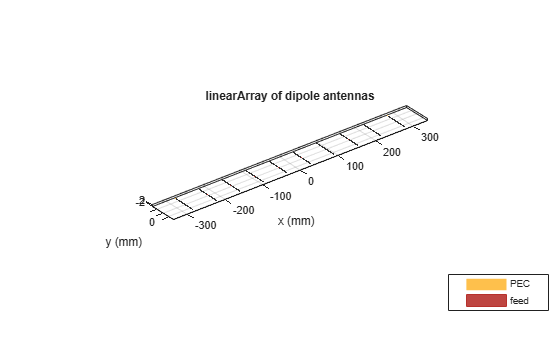# doa

Direction of arrival of signal

## Syntax

``[phiArrival,thetaArrival] = doa(obj,freq)``

## Description

example

````[phiArrival,thetaArrival] = doa(obj,freq)` calculates the direction of arrival of a signal when the transmission source is either a single-feed antenna defined using a `conformalArray` object or an incident plane-wave defined using a `planeWaveExcitation` object.```

## Examples

collapse all

Visualize a transmitter-receiver system as a conformal array. Use a `conformalArray` object with a dipole antenna as the transmitter element and an array of dipoles as the receiver element. Specify an operating fequency of 70 MHz.

```f = 70e6; lambda = 3e8/f;```

Specify the location coordinates of the transmitter and the receiver.

```txCenterOffset = [200,50,370]; rxCenterOffset = [0,0,0];```

Create the first and second element of the conformal array.

```elem1 = design(dipole,f); elem1.Tilt = 90; elem2 = linearArray('Element',elem1,'NumElements',4,'ElementSpacing',0.4*lambda);```

Create and view the conformal array.

```c = conformalArray; c.Element = {elem1 elem2}; c.ElementPosition = [txCenterOffset;rxCenterOffset];```

Calculate the direction of arrival.

`[phiArrival,thetaArrival] = doa(c,f)`
```phiArrival = 14.0362 ```
```thetaArrival = 27.9883 ```

Calculate the direction of arrival of a signal using the plane-wave excitation object. Use a linear array of dipoles operating at 2.4 GHz as the receiver element and set the azimuth and elevation reference angles.

```azimuthRef = 10; elevationRef = 30; f = 2.4e9; lambda = 3e8/f;```

Design a dipole antenna using the specified frequency.

```d = design(dipole,f); d.Tilt = 90;```

Create and view a linear array.

```ant = linearArray('Element',d,'NumElements',10,'ElementSpacing',0.5*lambda); show(ant)```Create a plane-wave excitation object using the dipole array.

```dir = [sind(elevationRef)*cosd(azimuthRef) sind(elevationRef)*sind(azimuthRef) -cosd(elevationRef)]; pol = [cosd(elevationRef)*cosd(azimuthRef) cosd(elevationRef)*sind(azimuthRef) sind(elevationRef)]; pw = planeWaveExcitation('Element',ant,'Direction',dir,'Polarization',pol);```

Calculate the direction of arrival.

`[phiArrival,thetaArrival] = doa(pw,f)`
```phiArrival = 10 ```
```thetaArrival = 29.8870 ```

## Input Arguments

collapse all

Transmitter-receiver system, specified as a `conformalArray` object or a `planeWaveExcitation` object.

To define the transmitter-receiver system as a conformal array of two elements, specify this input as a `conformalArray` object. The first element of the conformal array is a single-feed antenna, and is the transmission signal source. The second element of the conformal array is the receiver array.

To define the transmitter-receiver system as a plane-wave excitation, specify this input as a `planeWaveExcitation` object. The plane-wave defined using the `Direction` and `Polarization` properties of the `planeWaveExcitation` object is the incident signal. The linear or rectangular array defined using the `Element` property of the `planeWaveExcitation` object is the receiver.

Note

The `doa` function accepts only linear or rectangular array configurations for the receiver array of the `conformalArray` and `planeWaveExcitation` objects. The number of elements along each axis of the receiver array must be even, and the center of the receiver array must be at the origin.

Frequency of the incident signal in Hz. to use for the direction of arrival calculations, specified as a nonnegative scalar or a vector with nonnegative entries.

Example: `f = 70e6`

Data Types: `double`

## Output Arguments

collapse all

Azimuth angle of arrival of the signal in degrees, returned as a scalar or vector. The function calculates this output with reference to the center of the receiver array.

Data Types: `double`

Elevation angle of arrival of the signal in degrees, returned as a scalar or vector. The function calculates this output with reference to the center of the receiver array.

Data Types: `double`

## Algorithms

To estimate the direction of arrival, the `doa` function uses the fundamentals of RF propagation from the transmission to the reception of the signal. The phase of a far-field received signal ER(r) at an individual antenna is:

`${E}_{R}\left(r\right)\text{\hspace{0.17em}}\alpha \text{\hspace{0.17em}}{e}^{j\beta r}\text{\hspace{0.17em}}\text{\hspace{0.17em}}\left(1\right)$`

where β is the propagation constant of the received signal and r is the distance between the transmit and receive elements.

For a given transmit antenna, the position of the antenna element is [xtx, ytx, ztx], where

`${x}_{tx}=\rho \mathrm{cos}\left(\phi \right)\mathrm{sin}\left(\theta \right)\text{\hspace{0.17em}}\text{\hspace{0.17em}}\text{\hspace{0.17em}}\left(2\right)$`

`${y}_{tx}=\rho \mathrm{sin}\left(\phi \right)\mathrm{sin}\left(\theta \right)\text{\hspace{0.17em}}\text{\hspace{0.17em}}\text{\hspace{0.17em}}\left(3\right)$`

`${z}_{tx}=\rho \mathrm{cos}\left(\theta \right)\text{\hspace{0.17em}}\text{\hspace{0.17em}}\text{\hspace{0.17em}}\left(4\right)$`

where ρ is the distance between the transmit element and the geometrical midpoint of the receive antenna array. θ is the elevation angle or the angular position of the transmit element from the z-axis and φ is the azimuth angle that lies in the xy-plane.

Assume that:

• The receiver array is a homogenous linear or rectangular array with identical elements.

• The separation between two adjacent elements of the receiver array is uniform. For a linear array, the element spacing is dspace. For a rectangular array, the row spacing is drow and the column spacing is dcol.

• The receiver array has an equal number of elements along each axis. The number of elements along each axis of the array is even. For a linear array, the number of elements is Nelem. For a rectangular array, the number of elements in a row is Nrow and the number of elements in a column is Ncol.

For a uniform linear array (ULA) where the receive array elements lie along the x-axis, the position of the nth elements is

`${x}_{po{s}_{n}}=\frac{{d}_{space}}{2}\left({N}_{elem}+1-2n\right)\text{\hspace{0.17em}}\text{\hspace{0.17em}}\text{\hspace{0.17em}}\left(5\right)$`

where Nelem is the number of elements in the ULA.

For the uniform rectangular array (URA), which lies in the xy-plane, the position of the receive array element in the nth row and mth column is [xposn yposn], where

`${x}_{po{s}_{n}}=\frac{{d}_{col}}{2}\left({N}_{elem}+1-2n\right)\text{\hspace{0.17em}}\text{\hspace{0.17em}}\text{\hspace{0.17em}}\left(6\right)$`

`${y}_{po{s}_{n}}=\frac{{d}_{row}}{2}\left({M}_{elem}+1-2m\right)\text{\hspace{0.17em}}\text{\hspace{0.17em}}\text{\hspace{0.17em}}\text{\hspace{0.17em}}\left(7\right)$`

Consider a single-antenna transmitter located at (xtx, ytx, ztx). This equation determines the far-field separation r between the receiver array element and the transmitter for a ULA:

`${r}_{n}=\sqrt{{\left({x}_{tx}-{x}_{po{s}_{n}}\right)}^{2}+{\left({y}_{tx}\right)}^{2}+{\left({z}_{tx}\right)}^{2}}\text{\hspace{0.17em}}\text{\hspace{0.17em}}\text{\hspace{0.17em}}\text{(ULA)}\text{\hspace{0.17em}}\text{\hspace{0.17em}}\left(8\right)$`

.

This equation determines the far-field separation r between the transmitter and the element in the mth column and nth row of a URA:

`${r}_{m,n}=\sqrt{{\left({x}_{tx}-{x}_{po{s}_{n}}\right)}^{2}+{\left({y}_{tx}-{y}_{po{s}_{n}}\right)}^{2}+{\left({z}_{tx}\right)}^{2}}\text{\hspace{0.17em}}\text{\hspace{0.17em}}\text{\hspace{0.17em}}\text{(URA)}\text{\hspace{0.17em}}\text{\hspace{0.17em}}\left(9\right)$`

The function uses these equations to calculate the phase of the electric field by doing a full-wave electromagnetic simulation and uses this phase to calculate the direction of arrival in terms of the elevation and azimuth angles.

## Version History

Introduced in R2022a